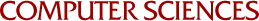## Algorithms for Polynomial Factorization

David R. Musser
1971

Algorithms for factoring polynomials with arbitrarily large integer coefficients into their irreducible factors are described. These algorithms are based on the use of mod p factorizations and constructions based on Hensel's Lemma. The concept of an "abstract algorithm" is defined and used in the development of general algorithms which are applicable in numerous polynomial domains, including polynomials with either integer or finite field coefficients. Included is a new generalization of Hensel's p-adic construction which is applicable to multivariate factorization. For the case of univariate polynomials over the integers, full specifications are given for algorithms which have been implemented and tested using the SAC-1 System for Symbolic and Algebraic Calculation. Theoretical computing times for the univariate algorithms are also derived and empirical data obtained during tests of the algorithms are reported.